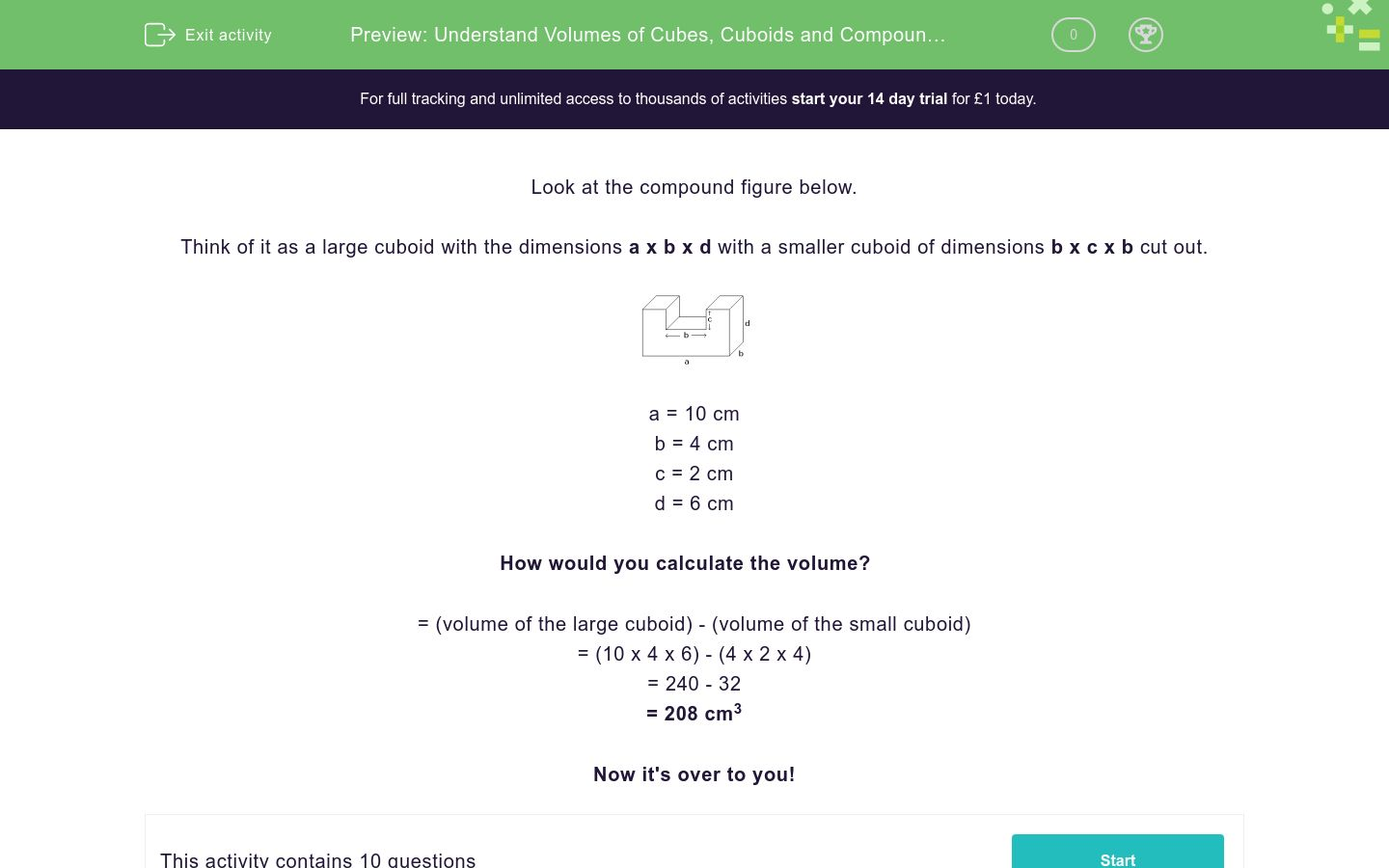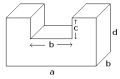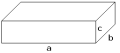# Understand Volumes of Cubes, Cuboids and Compound Figures

In this worksheet, students will calculate the volume of cubes, cuboids and compound figures.Key stage:  KS 3

Curriculum topic:   Geometry and Measures

Curriculum subtopic:   Use 3D Shape Knowledge to Solve Problems

Difficulty level:### QUESTION 1 of 10

Look at the compound figure below.

Think of it as a large cuboid with the dimensions a x b x d with a smaller cuboid of dimensions b x c x b cut out.a = 10 cm

b = 4 cm

c = 2 cm

d = 6 cm

How would you calculate the volume?

= (volume of the large cuboid) - (volume of the small cuboid)

= (10 x 4 x 6) - (4 x 2 x 4)

= 240 - 32

= 208 cm3

Now it's over to you!

 Find the volume of the following cuboid.a = 13 cm

b = 11 cm

c = 6 cm

Volume = ____ cm3

 Find the volume of the following cuboid.a = 11 cm

b = 9 cm

c = 5 cm

Volume = ____ cm3

 Find the volume of the following cuboid.a = 14 cm

b = 8 cm

c = 6 cm

Volume = ____ cm3

 Find the volume of the following compound figure.a = 10 cm

b = 12 cm

c = 6 cm

d = 10 cm

Volume = ____ cm3

336

236

319

380

 Find the volume of the following cube.c = 7 cm

Volume = ____ cm3

 Find the volume of the following compound figure, which is made of cuboids.a = 17 cm

b =12 cm

c = 4 cm

d =12 cm

Volume = ____ cm3

 Find the volume of the following compound figure, which is made of cuboids.a = 18 cm

b =12 cm

c = 6 cm

d = 7 cm

Volume = ____ cm3

 Find the volume of the following compound figure, which is made of cuboids.a = 14 cm

b = 11 cm

c = 7 cm

d = 8 cm

Volume = ____ cm3

 Find the volume of the following cuboid.a = 11.5 cm

b = 9 cm

c = 8 cm

Volume = ____ cm3

 Find the volume of the following compound figure.a = 10 cm

b = 12.5 cm

c = 6.5 cm

d = 10 cm

Volume = ____ cm3

232.375

235.475

234.375

236.375

• Question 1
 Find the volume of the following cuboid.a = 13 cm

b = 11 cm

c = 6 cm

Volume = ____ cm3

858
EDDIE SAYS
Volume = l x b x w = 13 x 11 x 6 = 858 cm³ Strong start! Is your working similar to the one above? Take your time and work through these questions step by step.
• Question 2
 Find the volume of the following cuboid.a = 11 cm

b = 9 cm

c = 5 cm

Volume = ____ cm3

495
EDDIE SAYS
Volume = l x w x b = 11 x 9 x 5 = 495 cm³ Don't forget the units, their really important if you want to gain all the marks available.
• Question 3
 Find the volume of the following cuboid.a = 14 cm

b = 8 cm

c = 6 cm

Volume = ____ cm3

672
EDDIE SAYS
Volume = l x b x w = 14 x 8 x 6 = 672 cm³ You're getting stronger with each question you attempt.
• Question 4
 Find the volume of the following compound figure.a = 10 cm

b = 12 cm

c = 6 cm

d = 10 cm

Volume = ____ cm3

336
EDDIE SAYS
In order to work out this volume of this figure you had to calculate: = (a x b x d) - (b x c x b) = (10 x 12 x 10) - (12 x 6 x 12) = (1200) - (864) = 336 cm³ How did you get on?
• Question 5
 Find the volume of the following cube.c = 7 cm

Volume = ____ cm3

343
EDDIE SAYS
All sides of a cube are the same. Volume = c x c x c = 7 x 7 x 7 = 343 cm³
• Question 6
 Find the volume of the following compound figure, which is made of cuboids.a = 17 cm

b =12 cm

c = 4 cm

d =12 cm

Volume = ____ cm3

1872
EDDIE SAYS
Volume = (a x b x d) - (b x c x b) = (2448) - (576) = 1872 cm³ Are these kinds of calculations of the volume beginning to get less daunting? You're beyond halfway, great work so far!
• Question 7
 Find the volume of the following compound figure, which is made of cuboids.a = 18 cm

b =12 cm

c = 6 cm

d = 7 cm

Volume = ____ cm3

864
EDDIE SAYS
Volume = (a x b x d) - (a x c x c) = 1512 - 648 = 864 cm³ These questions are getting more challenging but the formula remains the same, there are just added calculations.
• Question 8
 Find the volume of the following compound figure, which is made of cuboids.a = 14 cm

b = 11 cm

c = 7 cm

d = 8 cm

Volume = ____ cm3

546
EDDIE SAYS
Volume = (a x b x d) - (a x c x c) = (14 x 11 x 8) - (14 x 7 x 7) = 1232 - 686 = 546 cm³
• Question 9
 Find the volume of the following cuboid.a = 11.5 cm

b = 9 cm

c = 8 cm

Volume = ____ cm3

EDDIE SAYS
Volume = a x b x c = 11.5 x 9 x 8 = 828 cm³ Take a deep breath, you’ve got this. One more question to go.
• Question 10
 Find the volume of the following compound figure.a = 10 cm

b = 12.5 cm

c = 6.5 cm

d = 10 cm

Volume = ____ cm3

234.375
EDDIE SAYS
Volume = (a x b x d) - (b x c x b) = 1250 - 1015.625 = 234.375 cm³ Great work maths-whiz! That's another activity ticked off, phew.
---- OR ----

Sign up for a £1 trial so you can track and measure your child's progress on this activity.

### What is EdPlace?

We're your National Curriculum aligned online education content provider helping each child succeed in English, maths and science from year 1 to GCSE. With an EdPlace account you’ll be able to track and measure progress, helping each child achieve their best. We build confidence and attainment by personalising each child’s learning at a level that suits them.

Get started